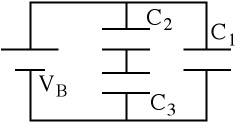# Three capacitors connected to a battery

• Vedj

## Homework StatementExamine the figure shown. Note that C3 = 10.5 uF, and C1 = C2 = 2C3. Also the battery voltage is Vb = 39.0 volts.

## Homework Equations

What is the equivalent capacitance for this arrangement?
How much charge is stored on C1?

## The Attempt at a Solution

C2 and C3 are in parallel, so I used C'=C2+C3
C' is in series with C1, so the total capacitance, C'' is:

((C1)(C'))/(C1+C')

which is equal to:

((C1)(C2+C3))/(C1+C2+C3)

pluging in values, I get an answer of 12.6 uF. I am using an online homework program and am told that this is incorrect. What am I doing wrong?

For the second question, shouldn't I multiply my answer for the first part by the volts value given?

Thank you for you help.

(c2+c3/2)+c1=C

c2 and c3 are in series, add up the values and divide by the number of caps then add that number to c1 and you have the total capacitance. second question, no, voltage has nothing to do with it.